##Rotating triangle's sides about their middle (2)

This continues the discussion started in RotatingSides.html .
Rotate the sides of triangle t = (ABC) about their middles, by an angle (phi). Extending them and taking intersections, defines a new triangle s = (GHI), similar to t. As the angle (phi) varies, the triangle s takes various positions and the following facts are true:
(1) When (phi) is equal to the Brocard angle w of triangle t, or -w, the corresponding symmedian point P of s coincides with the one or other Brocard point of t.
(2) The circumcircles d1, d2, d3 of triangles GAI, HBG and HCI respectively, have their radical axis coinciding with the symmedians of triangle s. Hence their radical center coincides with the symmedian point P of s.
(3) The 2nd Brocard triangle of t is similar to the triangle of centers of circles d1, d2 and d3 of (4).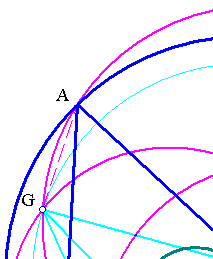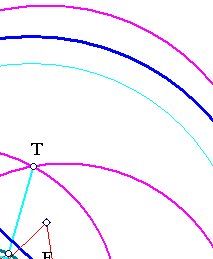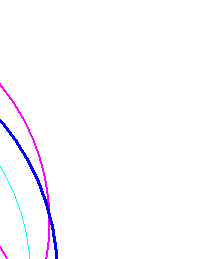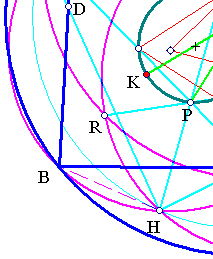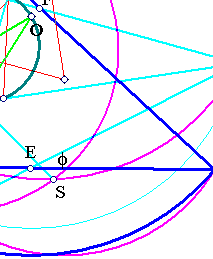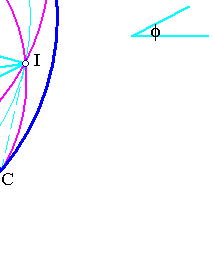The discussion in the previous reference, shows that triangle s = GHI is similar to triangle t = ABC, by a similarity centered at the circumcenter O of t and ratio equal to cos(phi). This proves the first statement. The second statement, on the radical axis relies on the proposition proved in the file SymmedianProperty.html . Having identified the symmedians with the radical axis, the triangle of centers of d1, d2, d3 is similar to the 2nd Brocard, since its sides are orthogonal to the symmedians.

The discussion continues in RotatingSides3.html , where it is shown that the triangle RST is homothetic to the [cosymmedian] triangle of GHI, which is similar to the cosymmedian triangle of t.

 Produced with EucliDraw© http://users.math.uoc.gr/~pamfilos/